### Vol 17, No 10 (2017) / Gambino

Updating the orbital ephemeris of the dipping source XB 1254–690 and the distance to the source

# Updating the orbital ephemeris of the dipping source XB 1254–690 and the distance to the source

Gambino Angelo F.1, , Iaria Rosario1, Di Salvo Tiziana1, Matranga Marco1, Burderi Luciano2, Pintore Fabio3, Riggio Alessandro2, Sanna Andrea2

Università degli Studi di Palermo, Dipartimento di Fisica e Chimica, via Archirafi 36 - 90123 Palermo, Italy
Università degli Studi di Cagliari, Dipartimento di Fisica, SP Monserrato-Sestu, KM 0.7, 09042 Monserrato, Italy
INAF-Istituto di Astrofisica Spaziale e Fisica Cosmica - Milano, via E. Bassini 15, I-20133 Milano, Italy

† Corresponding author. E-mail: angelofrancesco.gambino@unipa.it

Abstract: Abstract

XB 1254–690 is a dipping low mass X-ray binary system hosting a neutron star and showing type I X-ray bursts. We aim at obtaining a more accurate orbital ephemeris and at constraining the orbital period derivative of the system for the first time. In addition, we want to better constrain the distance to the source in order to locate the system in a well defined evolutive scenario. We apply, for the first time, an orbital timing technique to XB 1254–690, using the arrival times of the dips present in the light curves that have been collected during 26 yr of X-ray pointed observations acquired from different space missions. We estimate the dip arrival times using a statistical method that weights the count-rate inside the dip with respect to the level of persistent emission outside the dip. We fit the obtained delays as a function of the orbital cycles both with a linear and a quadratic function. We infer the orbital ephemeris of XB 1254–690, improving the accuracy of the orbital period with respect to previous estimates. We infer a mass of M 2 = 0.42 ± 0.04 M ʘ for the donor star, in agreement with estimations already present in literature, assuming that the star is in thermal equilibrium while it transfers part of its mass via the inner Lagrangian point, and assuming a neutron star mass of 1.4 M ʘ. Using these assumptions, we also constrain the distance to the source, finding a value of 7.6 ± 0.8 kpc. Finally, we discuss the evolution of the system, suggesting that it is compatible with a conservative mass transfer driven by magnetic braking.

Keywords: stars: neutron;stars: individual (XB 1254-690);X-rays: binaries;X-rays: stars;Astrometry and celestial mechanics: ephemerides

## 1 Introduction

XB 1254–690 is a persistent low mass X-ray binary (LMXB) showing type I X-ray bursts and dips. Coordinates of the source have been accurately derived from Iaria et al. () who, using one Chandra observation, located the source at RA (J2000) = 194.4048° and Dec (J2000) = −69.2886° with a 90% confidence level error box with radius 0.6′′. The dipping activity was revealed during an EXOSAT observation in 1984 (Courvoisier et al. ) and consists of a periodic decrease in the count-rate. This decrease is caused by photoelectric absorption in part of the X-ray emission by the cold (and/or partially ionized) bulge of matter that forms as a consequence of the impact of transferred plasma from the companion star onto the outer accretion disk (White & Swank ). Although type I X-ray bursts can be used to test the presence of a neutron star (NS) in the system (Mason et al. ), the presence of dips together with the absence of eclipses in the light curve constrains the inclination angle i of the system with respect to the line of sight to the observer between 60° and 80° (see Frank et al. ). The optical counterpart has been identified by Griffiths et al. () to be the faint V≃19 star GR Mus.

Timing analysis of the periodic dips led to an estimation of the orbital period of the system. Courvoisier et al. () estimated the orbital period of the system on the basis of the recurrence time of X-ray dips, obtaining a period of 0.162(6)d. Motch et al. () improved this estimate by giving a period of 0.163890(9)d obtained from optical data, while Díaz obtained an orbital period of 0.16388875(17)d, generating a periodogram from RXTE/All Sky Monitor (ASM) data. In their survey of the timing properties of several LMXB systems, instead, Levine et al. () assigned an orbital period of 0.1638890(4) d to XB 1254–690. Observations, however, revealed that the dipping activity is not always present and that the dips are quite different in shape from one observation to another.

Bhattacharyya () reported weak evidence of quasi periodic oscillations at about 95 Hz detected during one thermonuclear X-ray burst. Díaz, Trigo et al. (), based on the results of their spectral analysis performed on the source using XMM-Newton and INTEGRAL data, proposed the presence of a tilted accretion disk in the system. In this way they justified the disk temperature changes observed between the dip and non-dip time intervals of XMM/INTEGRAL light curves, as well as optical modulation observed with data collected from the Optical Monitor (OM) on XMM-Newton. The modulation of the optical light curve had already been observed by Motch et al. () These authors observed that the optical light curve shows minima occurring 0.15 in phase after the X-ray dips, suggesting that the modulation is due to the varying aspect of the X-ray heated atmosphere of the donor star. They, however, did not rule out the presence of an asymmetric accretion disk that does not completely shadow the companion star. A further confirmation of the hypothesis of a tilted accretion disk was proposed by Cornelisse et al. () They actually took advantage of the ephemeris from Díaz, revealing the presence of a negative superhump (i.e. a periodic photometric hump having a period shorter than the orbital period by a few percent). This supported the idea that XB 1254–690 could host a precessing accretion disk with a retrograde precession motion with a period of 6.74±0.07 d.

Cornelisse et al. () proposed that the disk is tilted out of the orbital plane along its line of nodes, implying that a large fraction of matter transferred from the companion star to the NS overflows or underflows the accretion disk instead of hitting the disk’s rim. In their opinion this could explain the presence of absorption features observed by Iaria et al. () and Boirin & Parmar (). They also found marginal evidence of a possible positive superhump suggesting that the accretion disk is possibly eccentric due to effects of tidal resonance. On the basis of these results they inferred the mass ratio of the system, q = M 2/M 1 = 0.33 − 0.36, and constrained the mass of the NS, M 1, between 1.2 and 1.8 M .

A first estimate of the distance was advanced by Courvoisier et al. (). Using EXOSAT data, they inferred the distance to the source from two type-I bursts, assuming that the luminosity at the burst peak was the Eddington luminosity for a 1.4 M NS. They obtained a distance of 12±2 kpc and 11±2 kpc for the two bursts, respectively. Subsequently, Motch et al. () constrained the distance to the source in the range 8−15 kpc from a modeling of the optical emission. Their modeling also showed that the optical brightness of the source is explained well when assuming that the donor star is near the main sequence. in’t Zand et al. () reported an estimation of the distance to the source by analyzing data collected from BeppoSAX in 1999. The detection of a possible photospheric radius expansion (PRE) during one superburst precursor allowed them to estimate a distance of 13±3 kpc. A successive work by Galloway et al. () allowed inferring an estimation of the distance, again on the basis of properties of type I X-ray bursts from the source. Analyzing data collected from the proportional counter array (PCA) on board the RXTE mission, Galloway et al. () found only marginal evidence of PRE during the observed type I X-ray bursts, and estimated a distance to the source of 15.5±1.9 kpc in the case of a companion star with cosmic abundances, and of 20±2 kpc in the case of a pure helium donor star.

In this work, we update the orbital ephemeris of XB 1254–690 using pointed observations collected by different space missions during a total time span of about 26 yr. We constrain the orbital period derivative for the first time, and we give a revised estimate of the distance to the source. We also discuss mass transfer in the system, suggesting that the system experiences a conservative mass transfer driven by magnetic braking of the companion star. The paper is structured as follows: in Section 2 we describe the data selection and reduction, in Section 3 we present the data analysis and results, and in Section 4 we discuss these results. A conclusion is provided in Section 5.

## 2 Observation and data reduction

To analyze the data on XB 1254–690, we take advantage of all the available pointed observations in X-ray archival data. However, no dip could be found in BeppoSAX, ASCA, ROSAT, Swift or Chandra observations. We therefore analyzed only the data collected by EXOSAT, Ginga, RXTE and XMM-Newton, which altogether span a temporal window of about 26 yr (from 1984 to 2010).

EXOSAT observed XB 1254–690 eight times between 1984 February 5 and 1985 April 15. We used the background-subtracted data products of the EXOSAT Medium Energy experiment (ME) in the energy range between 1 and 8 keV. We binned the light curves at 6 s. In addition, we performed barycentric corrections using the ftool earth2sun, using the coordinates of the source found by Iaria et al. (). These coordinates will be used hereafter for all our subsequent analyses.

XMM-Newton observed the source five times between 2001 January 22 and 2007 March 9 with the EPIC-pn camera. The selected observations have been performed in fast timing mode. We processed the dataset with the epproc tool of the Scientific Analysis System (SAS) v. 14.0.0. We extracted source events only from a box centered at the RAWX coordinate of the maximum in the photon distribution (RAWX = 37), and having a width of 15 RAWX. We extracted light curves using the evselect tool, selecting only events with PATTERN ≤4 (single and double pixel events) and FLAG = 0 to ignore spurious events. The light curves have been extracted between 0.5 and 10 keV with a bin time of 0.006 s. Barycentric corrections have been applied with the barycen tool.

The available observations performed from RXTE/PCA are sparsely distributed in a temporal window of about 13 yr (from 1997 to 2010). We used background-subtracted Standard 2 light curves covering the energy range 2 – 9 keV. The light curves have a bin time of 16 s and the barycentric corrections have been performed using the tool faxbary.

Ginga observed the binary system with the Large Area Counter (LAC) experiment on 1989 July 17 (ObsID 900802113648) and on 1990 August 3 (ObsID 900803061648). We use the background-subtracted light curves collected from the top layer of the detector covering the 2–17 keV energy band. The light curves have a bin time of 16 s and barycentric corrections have been applied with the ftool earth2sun.

## 3 Data analysis

To obtain the orbital ephemeris of XB 1254nnnnn–690 we need the arrival times of the dip as a function of time or of orbital cycle. For the first time we apply a timing technique to the arrival times of the dips in order to improve the ephemeris of the source. The ephemerides obtained so far for XB 1254–690 in the X-ray band have been based on periodograms generated from ASM data that have lower statistical confidence than the pointed observations we use in our analysis, which altogether span a temporal period of 26 yr.

To obtain the dip arrival times we have to take into account the fact that XB 1254–690 shows dips varying in shape from one orbital cycle to another. For this reason, we cannot fit the dip with a specific function since this implies the assumption that the dip always has the same shape (see Gambino et al. ; Iaria et al. , in preparation).

To address this issue, we take advantage of the method developed by Hu et al. () to parameterize the dipping behavior of XB 1916-053, and to systematically study its variation. The method can be applied both to dippers and eclipsing sources, and represents a powerful tool to obtain the dip arrival time for sources showing dips that are strongly variable in width and depth during different orbital cycles. The only constraint required for this method is that the observation of at least a complete dip is required Hu et al. ().

Therefore we selected all the available pointed observations in which single dips appear to be complete, collecting a total of 14 dips to analyze. In addition to these dips, the Ginga observations (ObsIDs 900802113648 and 900803061648) show five incomplete dips. Similarly, three RXTE observations (ObsIDs 60044-01-01-03, 60044-01-01-05 and 60044-01-01-08) show a total of three partial dips close in time, and the other two incomplete dips are visible in two RXTE observations (ObsIDs 95324-01-02-000 and 95324-01-02-00). We will take into account all these incomplete dips in the second part of the data analysis.

We report all the selected observations in Table 1. In each light curve we excluded all the type I X-ray bursts present, removing temporal intervals starting 5 s before the rise time and ending 100 s after the peak time of each burst. We implemented the method of Hu et al. () on the available complete dips to find the dip arrival times. For each of these light curves we distinguished between the dip and the persistent (non-dip) states, by roughly guessing the boundaries of the dip (see Table 2). Then, we identified the persistent count-rate of the source by fitting data points belonging to the persistent state with a linear function that minimizes the χ 2. Hereafter, the persistent count-rate level will be denoted by I 0. To obtain the dip arrival time in the light curve of each of the complete dips we have to average the times of each point in the dip, weighting them by the difference between the corresponding count-rate and that of the predicted persistent state I 0. Then, re-arranging the relation of Hu et al. (), the time elapsed from the beginning of the observation at which the dip occurs is given by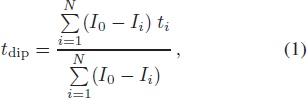where i is an integer index running from the left boundary (i = 1) to the right boundary (i = N) of the dip and Ii is the value of count-rate at each time ti included in the dip state domain. The procedure (applied on the dip of ObsID 405510401) is shown in Figure 1. From Equation (1) it is evident that the choice of the dip state boundaries (i = 1 and i = N) can be arbitrary. This is widely demonstrated by Hu et al. () with different tests, and could be naively explained by the fact that the points lying in the persistent state beside the dip give little contribution to the sum (i.e. I 0Ii ≃ 0), and hence to the determination of the time t dip at which the dip occurs.

Sequential n. Satellite/Instrument Observation ID Start time (UT) Stop time (UT) Number of dips
1 EXOSAT/ME 18332 1984 Feb 5 06:07:57 1984 Feb 5 09:08:14 1
2 EXOSAT/ME 31571 1984 Aug 7 03:10:27 1984 Aug 7 09:59:21 2
3 EXOSAT/ME 31593 1984 Aug 7 10:29:26 1984 Aug 7 14:04:14 1
4 EXOSAT/ME 49647 1985 Apr 15 04:27:23 1985 Apr 15 07:57:51 1
5 Ginga/LAC 900802113648, 900803061648 1990 Aug 02 11:37:52 1990 Aug 03 10:03:08 5
6 XMM/Epic-pn 60740101 2001 Jan 22 15:48:48 2001 Jan 22 20:02:19 1
7 RXTE/PCA 60044-01-01-02 2001 May 9 23:26:08 2001 May 10 01:37:04 1
8 RXTE/PCA 60044-01-01-03, 60044-01-01-05, 60044-01-01-08 2001 May 11 17:30:40 2001 May 12 11:46:40 3
9 XMM/Epic-pn 405510401 2007 Jan 14 01:12:04 2007 Jan 14 18:19:31 4
10 RXTE/PCA 93062-01-01-000 2008 Jan 16 05:28:00 2008 Jan 16 13:27:44 1
11 RXTE/PCA 95324-01-01-010 2009 Dec 31 03:51:12 2009 Dec 31 11:50:56 1
12 RXTE/PCA 95324-01-02-000, 95324-01-02-00 2010 Jan 1 01:44:48 2010 Jan 1 11:33:04 3
13 RXTE/PCA 95324-01-01-00 2009 Dec 31 21:01:52 2009 Dec 31 23:31:44 1

Table 1 Observations used for the Timing Analysis

Point Satellite/Instrument ObsID Time interval (s) Phase Interval Dip boundary (s) Dip boundary (Phase) t dip (s) ϕ dip
1 EXOSAT/ME 18332 0 – 13038 5400 – 6254 5895.3097
2 EXOSAT/ME 31571 0 – 12732 5500 – 9350 7170.5962
3 EXOSAT/ME 31571 15168 – 29328 18900 – 22950 21302.0361
4 EXOSAT/ME 31593 1044 – 15204 6600 – 10650 8423.0805
5 EXOSAT/ME 49647 3018 – 17178 8548 – 10748 9639.4763
6 Ginga/LAC 900802113648, 900803061648 0.55 – 1.55 0.91 – 1.13 1.04
7 XMM/Epic-pn 60740101 5542 – 19702 11301 – 13701 12259.9043
8 RXTE/PCA 60044-01-01-02 0 – 9368 300 – 3500 1917.2109
9 RXTE/PCA 60044-01-01-03, 60044-01-01-05, 60044-01-01-08 0.47 – 1.47 0.83 – 1.06 0.95
10 XMM/Epic-pn 405510401 1277 – 15437 8150 – 10051 9143.1486
11 XMM/Epic-pn 405510401 15462 – 29622 21800 – 23100 22526.2440
12 XMM/Epic-pn 405510401 30313 – 44473 36401.0, 38401.0 37425.4854
13 XMM/Epic-pn 405510401 45231 – 59391 50801 – 52901 52037.2646
14 RXTE/PCA 93062-01-01-000 0 – 10360 2500 – 4000 3235.2159
15 RXTE/PCA 95324-01-01-010 19352 – 33512 26205 – 26705 26409.6572
16 RXTE/PCA 95324-01-02-000, 95324-01-02-00 0.54 – 1.54 0.88, 1.20 1.05
17 RXTE/PCA 95324-01-01-00 56 – 14216 6610 – 8890 6990.6641
Notes: t dip is given in seconds from the start time of the corresponding observation. ϕ dip is the phase of arrival derived from the folding of close-in-time incomplete dips.

Table 2 Determination of the Arrival Times of Complete Dips and Phases of Folded Dips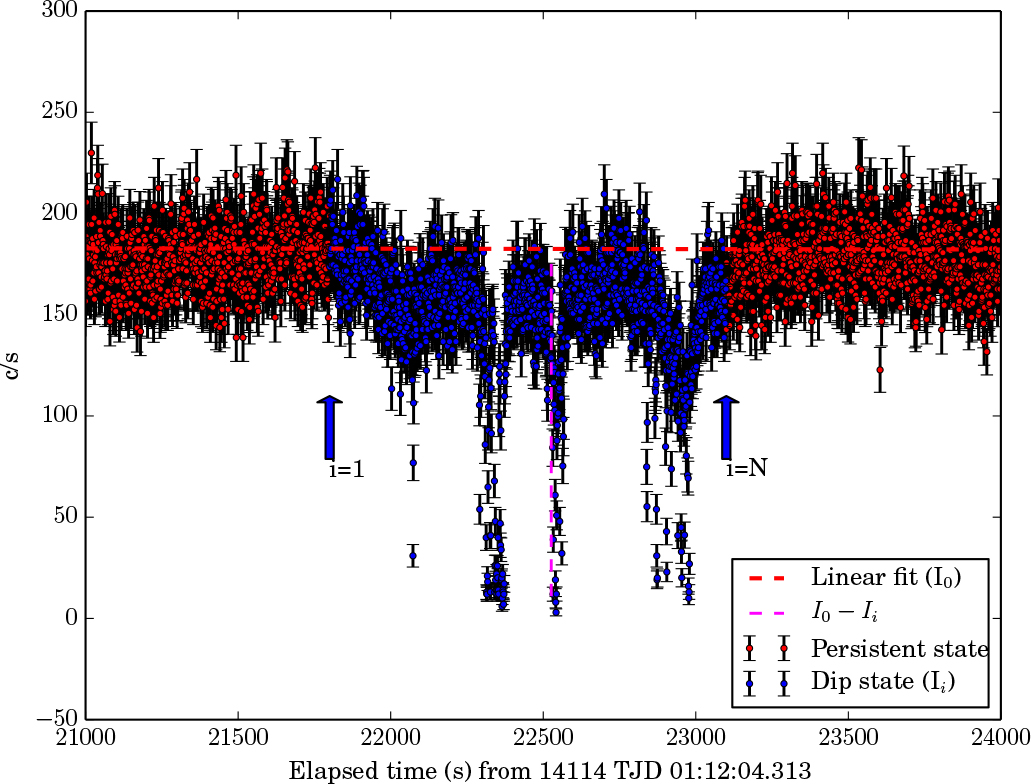Fig. 1 One of the dips (Point 11) analyzed in this work, corresponding to an observation (ObsID 405510401) performed by the XMM-Newton space mission. We indicate the division between the dip state (delimited between i = 1 and i = N) and the persistent state. The linear function fitting the persistent count-rate is shown as a red dashed line (I 0).

The arrival times of the dips are then calculated as T dip = T start + t dip, where T start is the starting time of the specific observation (see Table 1).We evaluate the delays of the observed dip arrival times with respect to the arrival times predicted using the orbital period P = 14160.01 s of Levine et al. () and an arbitrary reference epoch T 0 = 12733.0546TJD, corresponding to the arrival time of the dip observed in the XMM-Newton observation (ObsID 60740101). The arrival times, as well as the corresponding orbital cycle and the delays (OC), are reported in Table 3 (First Iteration).

First Iteration Second Iteration
Point Dip time (TJD;TDB) Cycle Delay (s) Dip time (TJD;TDB) Cycle Delay (s)
1 5735.324 –37809 202(544) 5735.324 –37809 –517(544)
2 5919.215 –36687 896(544) 5919.215 –36687 182(544)
3 5919.379 –36686 867(544) 5919.379 –36686 154(544)
4 5919.535 –36685 168(544) 5919.535 –36685 –545(544)
5 6170.297 –35155 1245(544) 6170.297 –35155 541(544)
6 11931.762 –23347 516(544)
7 11931.801 0 0(544) 11931.801 0 –493(544)
8 12038.999 654 1250(544) 12038.999 654 761(544)
9 11931.808 665 –728(544)
10 14114.156 13316 786(544) 14114.156 13316 373(544)
11 14114.311 13317 9(544) 14114.311 13317 –404(544)
12 14114.483 13318 748(544) 14114.483 13318 335(544)
13 14114.652 13319 1200(544) 14114.652 13319 787(544)
14 14481.265 15556 611(544) 14481.265 15556 211(544)
15 15196.466 19920 –306(544) 15196.466 19920 –680(544)
16 11931.844 19924 748(544)
17 15196.957 19923 –365(544) 15196.957 19923 –739(544)

Table 3 Journal of Arrival Times of the X-ray Dips Obtained from Each Light Curve for Both Iterations Performed in the Data Analysis

The error associated with the delays of the dip arrival times is determined by the standard deviation σ of the distribution of obtained phase delays associated with each dip. The σ of the distribution is equal to 0.04 which corresponds to 544 s according to the trial orbital period we used.

Using the same technique performed in Gambino et al. () and in Iaria et al. (), we fit the delays with a linear function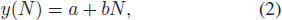where N is the number of orbital cycles, b is the correction to the trial orbital period (ΔP 0) in seconds and a is the correction to the trial reference time (ΔT 0) in seconds. We obtain χ 2(d.o.f.) = 12.94(12). The best-fit model parameters are reported in Table 4 (First Iteration). When applying the obtained corrections for the trial orbital period and the trial reference time, we find the following linear orbital ephemeris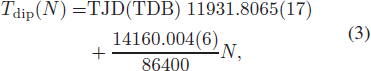where 11931.8065(17)TJD and 14160.004(6) s are the new reference time and orbital period, respectively. The associated errors are at the 68% confidence level.

First Iteration Second Iteration
a (s) 489 ± 154 954 ± 342 31±134 149±264
b (×10−3 s) –6 ± 6 –26±15 0±6 –5±11
c (×10−7 s) –9±6 –3±5

Table 4 Best-fit values obtained from the linear and quadratic fits to the delays in the dip arrival times.

However, we expect that, due to the orbital evolution of the binary system, a quadratic term has to be included in the orbital ephemeris. For this purpose, our method easily allows us to evaluate the orbital period derivative of the system by taking into account the possibility that delays follow a quadratic trend. Then, we fitted the delays with the quadratic function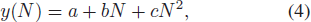where a is the reference time correction (ΔT 0) in seconds, b is the orbital period correction (ΔP 0) in seconds and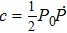in units of seconds. The best-fit parameters are provided in Table 4 (First Iteration) and allow us to obtain a new reference time T 0 of 11931.812(4)TJD, a new orbital period of 14159.984(15)s and an orbital period derivative = (−1.3 ± 2.0)× 10−10 s s−1. Here, the associated error on the orbital period is at the 68% confidence level, while the error on the orbital period derivative is at the 95% confidence level.

Nevertheless, this quadratic fit gives a χ 2(d.o.f.) = 10.73(11) and the estimated F-test probability of chance improvement with respect to the previous linear fit is 82%. This suggests that adopting the quadratic ephemeris does not significantly improve the fit.

The delays as a function of number of orbital cycles are shown in the upper panels of Figure 2. Superimposed we represent the best-fit linear function as a solid line. In the lower panel of the same figure we display residuals of the delays with respect to the linear best-fit function. The maximum deviation of the points with respect to the linear model is 794 s, that is 6% of the orbital period. Note, however, that the error we associate with the delays of the dip arrival times, while taking into account the statistical error produced by the photon counting and by the phase jitter, also includes the contribution of linear and quadratic terms, or even of higher orders of the time derivative of the orbital period. In order to avoid overestimating uncertainties on the fitting parameters, we again apply linear and quadratic fits, assuming the post-fit standard deviation has an error for each point. This error is determined by the distribution of points around the best fit parabolic trend. In this case, therefore, χ 2 cannot be used as an estimator of the goodness of fit, because the error is exactly equal to the distribution of points around the best-fit function, but in this way we get a correct estimate of the uncertainty of the fit parameters. The fits returned the same parameters we found previously and that are reported in Table 4. This means that the uncertainty in fit parameters is dominated in this case by large scattering intrinsic in the data.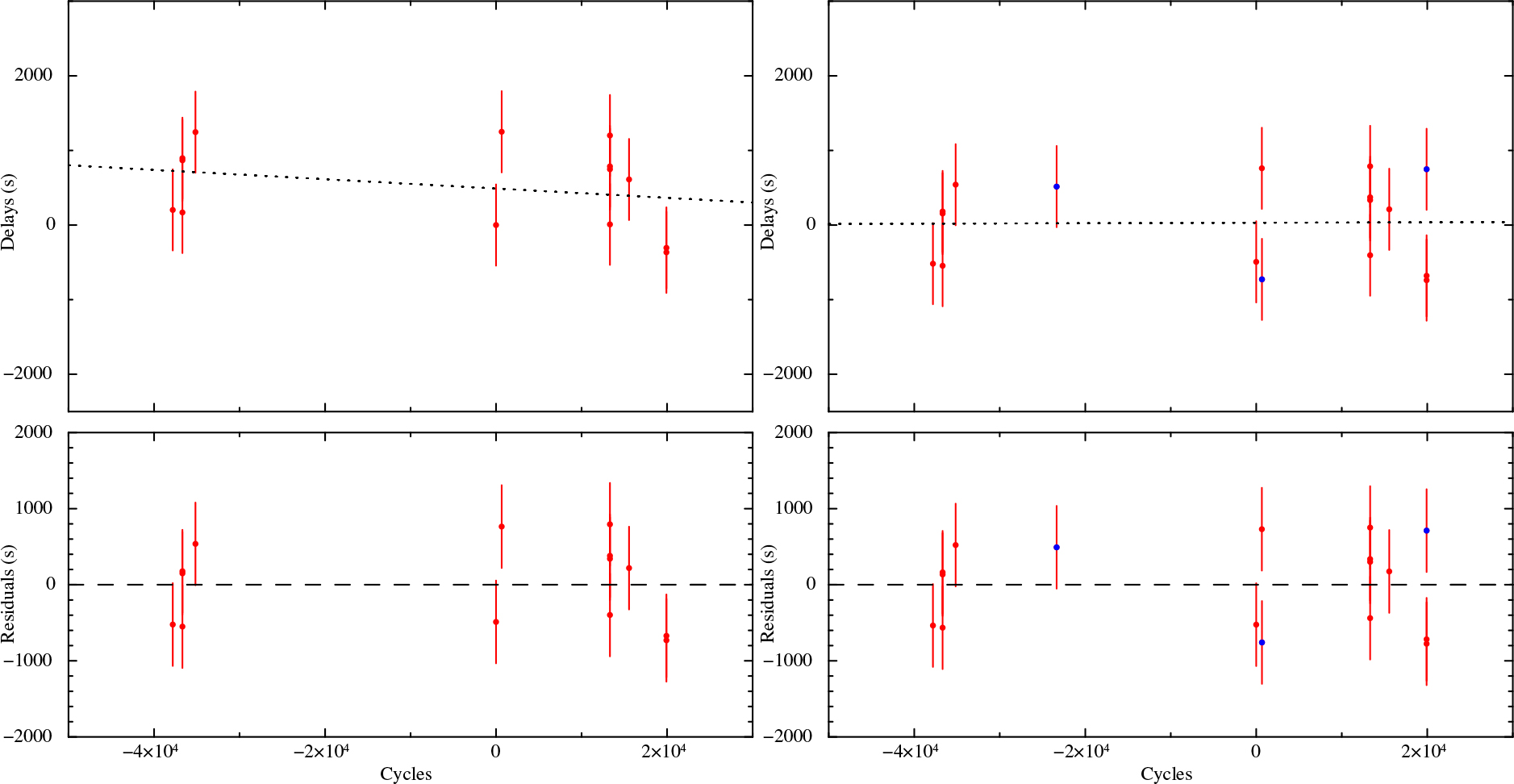Fig. 2 Delays as a function of orbital cycle for the first (left panels) and the second (right panels) iterations. The blue points represent the supplementary points added in the second iteration. Upper panels: linear fits of the delays. Lower panels: residuals of the delays with respect to the linear best-fit function.

In order to increase the statistics describing the timing technique, we can also take advantage of observations acquired by RXTE and Ginga (sequential numbers 5, 8 and 12 in Table 1) that we excluded in the first part of the analysis. These pointed observations, in fact, do not show a complete dip in the light curves, but if conveniently folded when close in time, they can provide further measurements of the dip arrival times.

We folded each group of these observations using the updated ephemeris given in Equation (3). As already done in the first part of the analysis, we distinguished the dip state from the persistent non-dip state by guessing the boundaries of the dip. Then, we applied the method of Hu et al. () to each folded dip profile, obtaining the phases at which the dips occur. The dip boundaries as well as the phases at which the folded dips occur are reported in Table 2.

The dip arrival times are estimated, starting from the obtained phases, as T dip = T 0 + (N + ϕ dip)P 0, where T 0 and P 0 are the reference epoch and the orbital period evaluated with the updated ephemeris of Equation (3) respectively. With this new trial orbital period P 0 we obtain the delays of the dip arrival times with respect to T 0. To be conservative with the first part of the analysis, we associate the same error with these delays that we evaluated for the first set of delays already analyzed during the first iteration.

In Table 3 (Second Iteration)we report the dip arrival times, the orbital cycles as well as the delays for these supplementary observations.

To integrate the delays evaluated in the first part of the analysis with those evaluated just now, we rescaled the delays obtained in the first iteration with respect to the new T 0 and P 0 of the updated ephemeris in Equation (3). We show the whole set of delays as a function of the corresponding orbital cycles in the right panel of Figure 2. We fitted all the delays with respect to the linear function in Equation (2), obtaining χ 2(d.o.f.) = 17.47(15). We list the best-fit model parameters in Table 4 (Second Iteration). Applying corrections suggested by the linear fit, we find the following new linear orbital ephemeris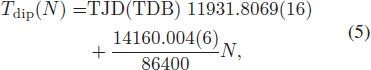where 11931.8069(16) TJD and 14160.004(6) s are the newly corrected reference epoch and orbital period, respectively. As done before, we also tried to fit the delays with the quadratic function in Equation (4). Also in this case, the best-fit parameters are provided in Table 4 (Second Iteration). Applying the corrections returned by the quadratic fit to the starting ephemeris, we obtain a new reference time T 0 of 11931.808(3)TJD, a new orbital period of 14160.000(11) s and an orbital period derivative = (0.0 ± 1.4) × 10−10 s s−1. The associated error on the orbital period is at the 68% confidence level, while the error on the orbital period derivative is at the 95% confidence level.

Nevertheless, the quadratic fit gives a χ 2(d.o.f.) = 17.20(14) and the estimated F-test probability of chance improvement with respect to the previous linear fit is 63%. This suggests that by adopting the quadratic ephemeris we do not significantly improve the fit.

Again, we ran the same fits using the post-fit standard deviation as error for each delay, obtaining compatible results.

Even though the new ephemeris of Equation (5) does not significantly improve the ephemeris of Equation (3), they are extended on a data set that includes all the available pointed observations in the X-ray archive. In addition, although the quadratic ephemeris does not improve the significance of the fit, it is fundamental to evaluate an upper limit on the orbital period derivative. The one reported in this paper also represents the only available constraint on this orbital period derivative present to date. This constraint will be improved when further observations of XB 1254–690 will be available.

## 4 Discussion

We derived and improved the orbital ephemeris for XB 1254–690 by taking advantage of the whole X-ray data archive, which consists of pointed observations spanning 26 yr. The direct measurement of the dip arrival times allowed us to increase the accuracy of the orbital period of the system by a factor of 10 with respect to the previous estimation of Levine et al. (), and by a factor of 4 with respect to the value estimated by D´ıaz Trigo et al. (). Furthermore, we evaluated, for the first time, a constraint on the orbital period derivative of | | < 1.4 × 10−10 s s−1. This value is compatible with zero and includes both positive and negative values and for this reason should be considered an upper limit on the modulus of the orbital period derivative. However, the result represents the first evaluation of this orbital parameter so far in the literature for this source and will be certainly improved by including future observations when these become available.

In the following, using the equations for secular evolution of the source, we discuss the mass transfer for XB 1254–690 in order to get more information on the system. As a first step, we evaluate the observed mass accretion rate onto the NS surface.

Iaria et al. (), modeling the spectrum of XB 1254–690 collected by BeppoSAX in 0.1–100 keV, estimated an averaged unabsorbed flux of 1.4×10−9 erg cm−2 s−1. Taking into account the value of distance of 15.5±1.9 kpc proposed by Galloway et al. () and the value of flux inferred by Iaria et al. (), and assuming an NS radius RNS of 10 km, we can estimate the observed mass accretion rate onto the NS as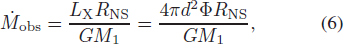where L X is the observed bolometric X-ray luminosity, M 1 is the mass of the NS that we assumed to be 1.4 M and Φ is the flux observed by Iaria et al. (). We obtain an observational mass accretion rate of (3.4±0.8)× 10−9 M yr−1

To understand in which evolutive scenario the system has to be located, we compare the observed mass accretion rate with that predicted by the theory of secular evolution for LMXB systems. As mechanisms of angular momentum loss, we take into account the emission of gravitational waves (gravitational radiation), as well as magnetic braking. We do not rule out the possibility that the magnetic braking term plays a role as a mechanism of angular momentum loss, owing to the fact that the mass of the companion star, inferred assuming the condition of thermal equilibrium (see eq. (25) in Verbunt ), is large enough to generate the dynamo effect resulting in a net magnetic field anchored into the companion star surface (see Nelson & Rappaport ).

The mass accretion rate predicted by the theory of secular evolution, under the assumed hypothesis, is given by the relation of Burderi et al. () (see also di Salvo et al. )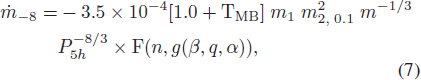where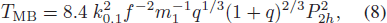where f is a dimensionless parameter of order unity: preferred values are f = 0.73 (Skumanich ) or f = 1.78 (Smith et al. ), and k 0.1 is the radius of gyration of the star in units of 0.1 (Claret & Gimenez ). Here, revealing in advance one result of the subsequent analysis, we will assume that the companion star is a main sequence star that has a mass of M 2 = 0.42 M . This is the mass it should have in order to fill its Roche Lobe for the orbital period of the system, ~ 3.9 h (see eq. (25) in Verbunt ).

We can compare the theoretical mass accretion rate predicted by Equation (7), adopting a conservative mass transfer scenario (β = 1), with the observed mass accretion rate in the source.

Adopting a distance to the source of d = 15.5±1.9 kpc (Galloway et al. ), and setting the parameter f in Equation (8) equal to 0.73 (Skumanich ), we observe an accordance between theory and observations at n ~ 0.8 (see Fig. 3, upper-left panel). This means that according to the theory of secular evolution in X-ray binary systems, we expect that the companion star is in thermal equilibrium. For the same distance, we also tried the parameter f = 1.78 (Smith et al. ) for magnetic braking, concluding that there is neither accordance with n = 0.8 nor with n = −1/3. Furthermore, we explored the case in which the magnetic braking term of Equation (8) does not play a role in angular momentum losses of the binary system. In this case, however, we do not observe any accordance between the theoretical mass accretion rate and that observed by Iaria et al. (), owing to the fact that in this case the theoretical mass accretion rate underestimates the observed mass accretion rate for any value of n.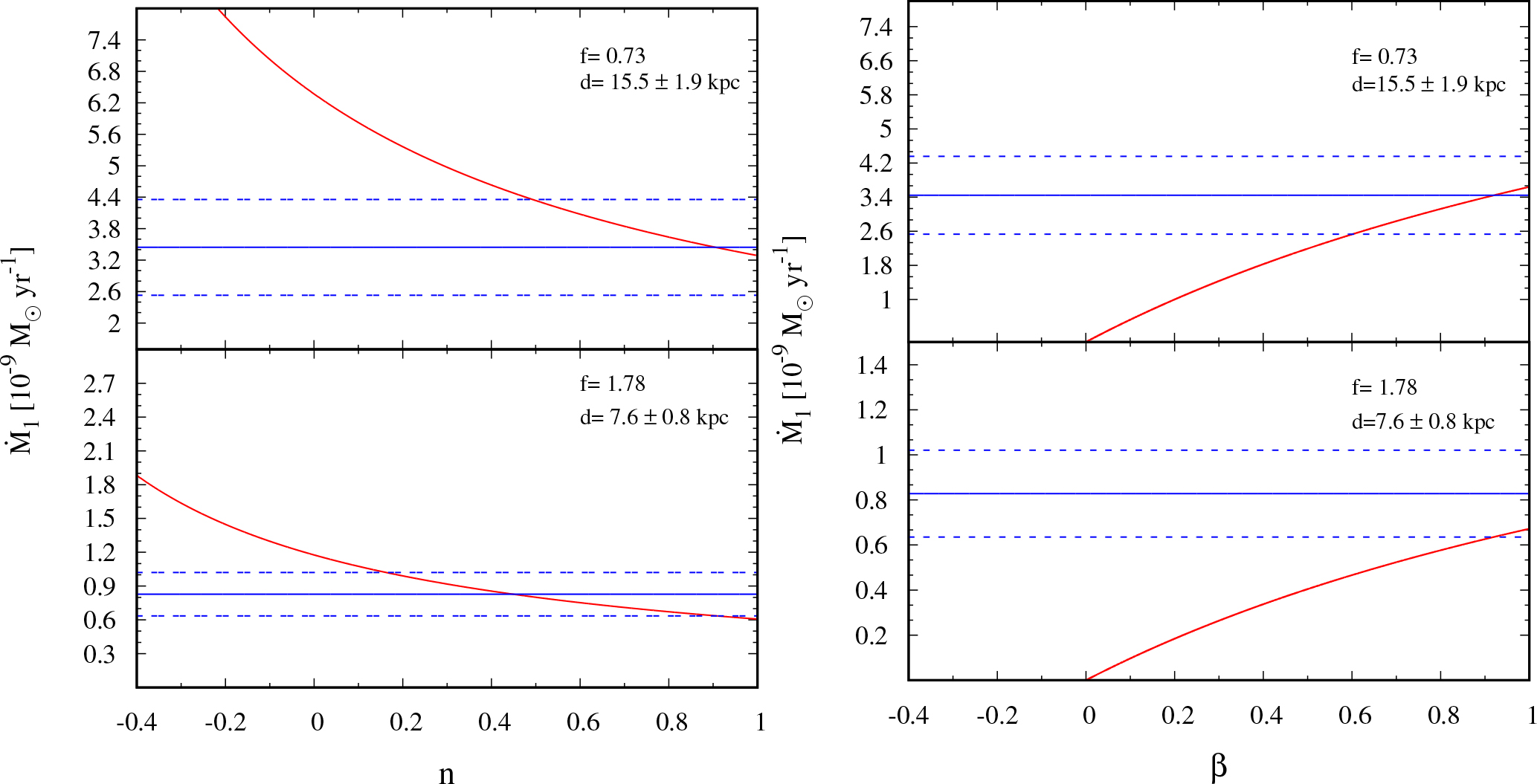Fig. 3 Red curves: theoretical mass accretion rate onto the NS surface predicted by Eq. (7). Blue lines: best value of the mass accretion rate obtained from the observations (solid lines) and relative errors (dashed lines) for d = 15.5±1.9 kpc and 7.6±0.8 kpc (upper and lower panels, respectively). Left plots: mass accretion rate as a function of n adopting a conservative mass transfer scenario (β = 1). Right plots: mass accretion rate as a function of β adopting an index n = 0.8 (donor star in thermal equilibrium).

This value of distance, however, as well as the distance of d = 13±3 kpc derived by in’t Zand et al. (), has been inferred by the analysis of X-ray type-I bursts, assuming that they show PRE, and thus that the peak luminosity of the bursts is the Eddington luminosity for an NS of 1.4M . However, as the same authors reported in their works, the observations they analyzed do not have enough statistics to be sure if a PRE occurred, and as a consequence of this their distances could be overestimated.

On the other hand, Cornelisse et al. () obtained an estimation of the range of q and of MNS, totally independent from assumptions about distance to the source. This allows us to obtain a range of masses for the donor star of 0.46–0.50M assuming a mass of the NS of 1.4M

To sketch the most probable evolutive scenario, as well as to constrain the distance of XB 1254–690, we need to evaluate the companion star mass using the results of our timing analysis. For this purpose, we assume that the companion star is a main sequence star in thermal equilibrium. The self-consistency of this hypothesis will be tested in a subsequent part of this discussion. All the available X-ray data of XB 1254–690 clearly demonstrate that the source is a persistent X-ray emitter over a temporal window of about 26 yr. As a consequence of this, we can properly assume that the companion star fills its Roche lobe, continuously transferring part of its mass to the NS. Thus, we impose that the companion star radius R 2 has to be equal to the Roche lobe radius R L2, given by the expression of Paczyǹnski ()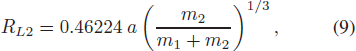where m 1 and m 2 are the NS and companion star masses in units of solar masses and a is the orbital separation of the binary system. Hereafter, we are going to assume an NS mass of 1.4 M for the subsequent analysis. Our assumption that the companion star belongs to the lower main sequence leads us to adopt the mass-radius relation of Neece () for M-stars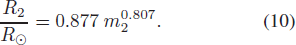Using Equation (9) and Equation (10) along with Kepler’s third law, which links the orbital separation a with the value of orbital period found with the linear ephemeris, we obtain a mass of 0.42±0.04 M for the donor star. Here we take into account an accuracy of 10% in the mass estimation (see Neece ).

Our estimation of the donor star mass of 0.42±0.04 M⊙ is in accordance with the values of Cornelisse et al. () and as a consequence of this, we guess that the donor is probably a main sequence star in thermal equilibrium. With this assumption, we can estimate the maximum distance that the system can have, assuming that the Kelvin-Helmholtz timescale τ KH (i.e. the characteristic time that a star spends to reach thermal equilibrium) is equal to the mass transfer timescale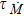.

The Kelvin-Helmholtz timescale is given by the relation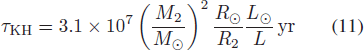of Verbunt (), where we adopt the mass-luminosity relation for M-type stars of Neece ()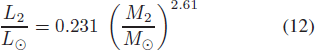and the mass-radius relation of Neece () in Equation (10).

On the other hand, the mass transfer timescale is given by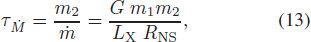where L X is the bolometric source luminosity.

Imposing the similarity between τ KH and, we obtain the maximum luminosity of the system under the hypothesis that the donor star is in thermal equilibrium on the main sequence: L X ~ (10 ± 2) × 1036 erg s−1.

The distance to the source for XB 1254–690 can be obtained by the flux inferred by Iaria et al. () in the band 0.1–100 keV and from the X-ray luminosity just obtained as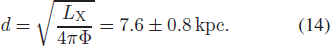Once we obtained the new value of distance, we repeated the comparison between mass accretion rate predicted by the theory of secular evolution and that observed by Iaria et al. (), rescaled for the updated distance.

In this case we observe that when assuming β = 1 (conservative mass transfer) there is no agreement between theory and observation adopting the parameter f = 0.73 in Equation (8). Using f = 1.78, however, the agreement is achieved for n ~ 0.8 (see Fig. 3, lowerleft panel). In this case, therefore, we find a solution that is consistent with our initial hypothesis of a donor star in thermal equilibrium. Again the magnetic braking term T MB is necessary to explain the observed mass accretion rate, owing to the fact that otherwise for every n no accordance is found between the theoretical mass accretion rate and the observed one.

For completeness, we also explore the nonconservative mass transfer scenario for both distances considered above, adopting the same f parameters used for the conservative case and assuming n = 0.8. For a distance of 15.5±1.9 kpc the observation is in agreement with the theory for a value of β ~0.9, i.e. 90% of the mass of the companion star is accreted onto the NS surface. On the other hand, adopting a distance of 7.6±0.8 kpc we find a lower limit for β of about 0.92. This actually means that most of the mass transferred bythe donor star is accreted onto the NS.

In order to have a further confirmation of the scenario just depicted for XB 1254–690, and to understand the temporal evolution that the orbital separation of the system will undergo, we use the relation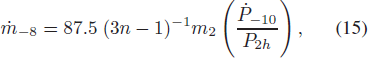of Burderi et al. () to obtain a theoretical estimation of the orbital period derivative of the system. In the equation, −10 is the orbital period derivative in units of 10−10 s s−1, P 2h is the orbital period of the system in units of two hours and −8 is the secondary mass derivative in units of 10−8 M yr−1. Adopting the value of at n = 0.8, the mass of the companion star inferred in our analysis and the orbital period obtained with the linear ephemeris, we predict a negative of −5× 10−13 s s−1, meaning that the binary system is expected to shrink in agreement with the assumption of a companion star in thermal equilibrium and with the assumed mass-radius index of 0.8.

The theoretical value of we inferred for this system is indeed compatible with the upper limit of the orbital period derivative obtained through the quadratic ephemeris: || < 1.4× 10−10 s s−1.

## 5 Conclusions

In this work we update the existent orbital ephemerides for XB 1254–690, taking advantage of about 26 yr of Xray data and performing direct measurements of the dip arrival times on different pointed observations. We further increase the accuracy reached by Levine et al. () by one order of magnitude.

The quadratic ephemeris, even though not statistically significant with respect to the linear ephemeris, allows us to constrain the orbital period derivative of the system for the first time. Assuming that the companion star is in thermal equilibrium, we infer a donor star mass of 0.42±0.04 M , which is in agreement with the range of masses estimated by Cornelisse et al. (), assuming that the mass of the NS is 1.4 M . In our analysis, we propose a different estimate of the distance to the source with respect to those obtained by in’t Zand et al. () and Galloway et al. (). These authors provided estimates of the distance to the source that, as they state, should be considered as upper limits to the distance to the source, owing to the relatively poor statistics of the data they analyzed. We suggest a new distance of 7.6±0.8 kpc that represents the distance for which the companion star has a Kelvin-Helmholtz timescale that is similar to the mass transfer timescale in this system. For larger distances, the inferred mass accretion rate would be higher, implying a mass transfer timescale shorter than the Kelvin-Helmholtz timescale of the donor, bringing the companion star out of thermal equilibrium.

These results, as well as the assumption of an NS of 1.4 M , allow us to state that the most probable scenario for this system is the one in which the companion star is in thermal equilibrium and that most of (if not all) the mass transferred by the companion is accreted onto the NS in a conservative way through the inner Lagrangian point, regardless of whether we assume a distance of 15.5±1.9 kpc or of 7.6±0.8 kpc. Moreover, the analysis strongly supports the idea that the magnetic braking plays an important role in the angular momentum loss in this binary system. In the hypothesis that the companion star is in thermal equilibrium with a mass-radius index of about 0.8, we also predict that the binary orbit is shrinking at a rate of about −5 × 10−13 s s−1. This prediction can be easily tested with future observations, when the uncertainty on the orbital period derivative we have now will be greatly reduced.

 Bhattacharyya S. 2007 MNRAS 377 198 Boirin L. Parmar A. N. 2003 A & A 407 1079 Burderi L. Di Salvo T. Riggio A. et al. 2010 A & A 515 A44 Claret A. Gimenez A. 1990 Ap & SS 169 215 Cornelisse R. Kotze M. M. Casares J. Charles P. A. Hakala P. J. 2013 MNRAS 436 910 Courvoisier T. J.-L. Parmar A. N. Peacock A. Pakull M. 1986 ApJ 309 265 di Salvo T. Burderi L. Riggio A. Papitto A. Menna M. T. 2008 MNRAS 389 1851 Díaz Trigo M. Parmar A. N. Boirin L. et al. 2009 A & A 493 145 Frank J. King A. R. Lasota J.-P. 1987 A & A 178 137 Galloway D. K. Muno M. P. Hartman J. M. Psaltis D. Chakrabarty D. 2008 ApJS 179 360 Gambino A. F. Iaria R. Di Salvo T. et al. 2016 A & A 589 A34 Griffiths R. E. Gursky H. Schwartz D. A. et al. 1978 Nature 276 247 Hu C.-P. Chou Y. Chung Y.-Y. 2008 ApJ 680 1405 Iaria R. Di Salvo T. Burderi L. Robba N. R. 2001 ApJ 548 883 Iaria R. di Salvo T. Lavagetto G. D’Aí A. Robba N. R. 2007 A & A 464 291 Iaria R. Di Salvo T. Gambino A. F. et al. 2015 A & A 582 A32 Iaria R. Gambino A. F. Di Salvo T. et al. 2017 arXiv:1703.05294 in’t Zand J. J. M. Kuulkers E. Verbunt F. Heise J. Cornelisse R. 2003 A & A 411 L487 Levine A. M. Bradt H. V. Chakrabarty D. Corbet R. H. D. Harris R. J. 2011 ApJS 196 6 Mason K. O. Seitzer P. Tuohy I. R. et al. 1980 ApJ 242 L109 Motch C. Pedersen H. Courvoisier T. J.-L. Beuermann K. Pakull M. W. 1987 ApJ 313 792 Neece G. D. 1984 ApJ 277 738 Nelson L. A. Rappaport S. 2003 ApJ 598 431 Paczyński B. 1971 ARA & A 9 183 Skumanich A. 1972 ApJ 171 565 Smith S. E. Noah P. V. Cottrell M. J. 1979 PASP 91 775 Verbunt F. 1993 ARA & A 31 93 White N. E. Swank J. H. 1982 ApJ 253 L61
Cite this article: Gambino Angelo F., Iaria Rosario, Di Salvo Tiziana, Matranga Marco, Burderi Luciano, Pintore Fabio, Riggio Alessandro, Sanna Andrea. Updating the orbital ephemeris of the dipping source XB 1254–690 and the distance to the source. Cancer Biol Med. 2017; 10:108.

### Refbacks

• There are currently no refbacks.